# Express the following rational numbers as decimals

Question:

Express the following rational numbers as decimals:

(i) $\frac{2}{3}$

(ii) $-\frac{4}{9}$

(iii) $\frac{-2}{15}$

(iv) $-\frac{22}{13}$

(v) $\frac{437}{999}$

(vi) $\frac{33}{26}$

Solution:

(i) Given rational number isNow we have to express this rational number in decimal form. So we will use the long division method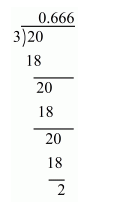Therefore $\frac{2}{3}=0.6666$

$\Rightarrow \frac{2}{3}=0 . \overline{6}$

Hence, $\frac{2}{3}=0 . \overline{6}$

(ii) Given rational number isNow we have to express this rational number into decimal form. So we will use long division method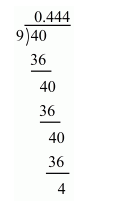Therefore, $\frac{4}{9}=0.444$

$\Rightarrow-\frac{4}{9}=-0 . \overline{4}$

Hence, $-\frac{4}{9}=-0 . \overline{4}$

(iii) Given rational number isNow we have to express this rational number into decimal form. So we will use long division method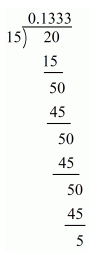Therefore $\frac{2}{15}=0.1333$

$\Rightarrow \frac{2}{15}=0.1 \overline{3}$

Hence, $-\frac{2}{15}-0.1 \overline{3}$

(iv) Given rational number isNow we have to express this rational number into decimal form. So we will use long division method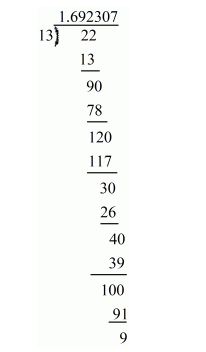Therefore $\frac{22}{13}=1.692307 \ldots$

$\Rightarrow \frac{22}{13}=1 . \overline{692307}$

Hence, $-\frac{22}{13}=-1 . \overline{692307}$

(v) Given rational number isNow we have to express this rational number into decimal form. So we will use long division method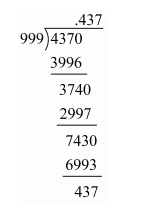$\Rightarrow \frac{437}{999}=0 . \overline{437}$

Hence, $\frac{437}{999}=0 . \overline{437}$

(vi) Given rational number isNow we have to express this rational number into decimal form. So we will use long division method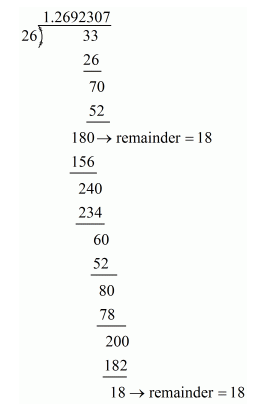Therefore $\frac{33}{26}=1.2 \overline{692307} \ldots$

Hence, $\frac{33}{26}=1.2 \overline{692307}$